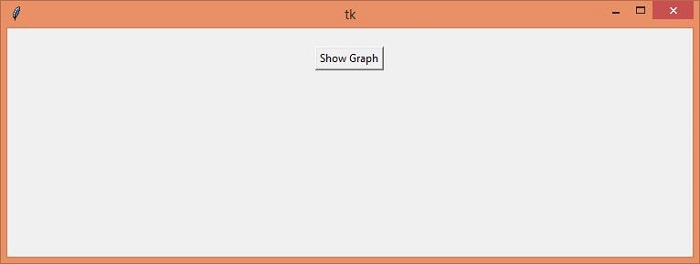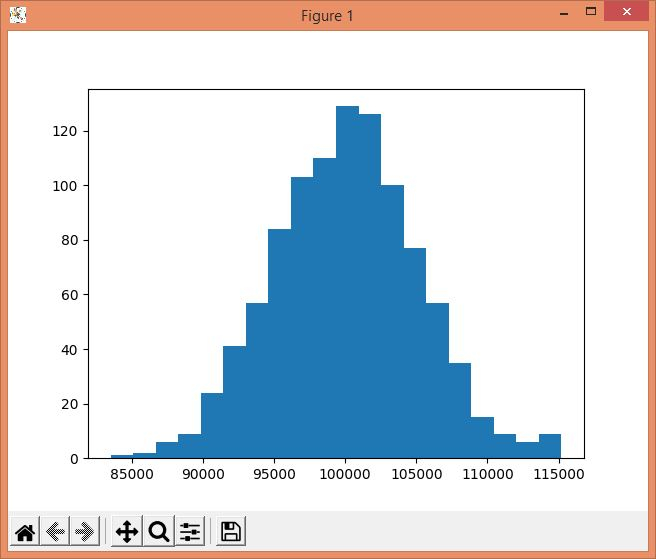# Placing plot on Tkinter main window in Python

Oftentimes, we need to deal with plots in our Tkinter GUI-based application. To support the plots for the available data points, Python provides a Matplotlib package that can be imported into the application easily. In order to add a plot for the given data points, we have to install several other packages such as NumPy along with Matplotlib. NumPy is a Python library that helps to deal with scientific calculation in the Data.

## Example

In this example, we will create data points for the car prices starting from (100000) with the units in the range of 1000 to 5000.

#Import the required Libraries
from tkinter import *
import numpy as np
import matplotlib.pyplot as plt

#Create an instance of Tkinter frame
win= Tk()

#Set the geometry of tkinter frame
win.geometry("750x250")

def graph():
car_prices=np.random.normal(100000, 5000, 1000)
plt.hist(car_prices, 20)
plt.show()

#Create a button to show the plot
Button(win, text= "Show Graph", command= graph).pack(pady=20)
win.mainloop()

## Output

If we will run the above code, it will display a window that contains a button "Show Graph".When we click the button, it will display the graph on the screen.